Next: Polynomial iterators. Up: Partial inverse heuristic for Previous: Convergence of partial inverses

It is easy to see that we can create an infinite number of iterators. For instance, for any iterator x = F(x), and arbitrary a,will also be fixed point iterator giving the same solution set for any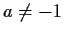.

A natural question to ask is, which iterator is better? This question is nontrivial to answer, and contradicts the spirit of the heuristic, which is to try all of them. We would like to try a large number of formulas and not conjecture which is the best one and use it alone. Yet an intuitive and very economic measure of the quality of the iterators is, the number of occurrences of xon the right-hand side of the iterator. A smaller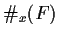is considered better. The degenerate case is a good example. If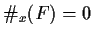, we have an explicit expression for the root. Using iterators which have the minimalguarantees, to a certain degree, that we will not be generating more complicated trivial iterators.

If at any point during our isolation or after trial simplification, the resulting equation has a smaller number of occurrences of x, we will derive all possible iterators arising from this shortened equation. For example,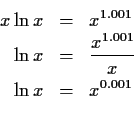The grouping of powers caused a simplification and from this equation we obtain two iterators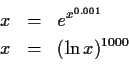The first one was obtained before but the second one is new and in this case it is useful to find the largest of the roots of the original equation.

The use ofas a measure of iterator quality seems to work in practice, although we cannot prove this generally. Certainly there are examples of two iterators for the same equation, where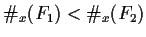, and F1 behaves worse than F2.Next: Polynomial iterators. Up: Partial inverse heuristic for Previous: Convergence of partial inverses
Gaston Gonnet
1998-07-08